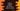# Python program to replace all negative numbers with zero in a list## How to replace all negative numbers with zero in Python list:

In this post, we will learn how to replace all negative numbers with zero in a Python list. We will learn different ways to do that.

In the first method, I will show you how to do that with a loop. Then we will move to some different methods like recursive to do that.

## Method 1: Replace all negative numbers with zero in a Python list by using a loop:

Let’s try this by using a loop first. Below is the complete program:

``````given_list = [1, 2, 3, 4, -1, -4, 5, -6]

print(f'List before changes: {given_list}')

for i in range(len(given_list)):
if given_list[i] < 0:
given_list[i] = 0

print(f'List after changes: {given_list}')``````

Here,

• given_list is the given list of numbers that includes both positive and negative values.
• We are printing this list before and after the changes are made.
• The for loop is used to update the list, i.e. assigning 0 to all negative numbers.
• It iterates through the list elements one by one.
• If it finds any value less than 0, it changes that value to 0.

If you run the above program, it will print the below output:

``````List before changes: [1, 2, 3, 4, -1, -4, 5, -6]
List after changes: [1, 2, 3, 4, 0, 0, 5, 0]``````

As you can see here, all negative numbers are changed to 0 in the list.

## Method 2: Replace all negative numbers with zero in a Python list recursively:

We can also do it recursively. A method is called recursive if it calls itself again and again to reach the final result.

So, we will write one recursive method that will call itself again and again and iterate through all numbers in the list. While iterating, it will update the numbers which are less than 0.

Below is the complete program that uses a recursive method for the same task:

``````def update_list(l, current_index):
if current_index == len(l):
return

if l[current_index] < 0:
l[current_index] = 0

update_list(l, current_index+1)

given_list = [1, 2, 3, 4, -1, -4, 5, -6]

print(f'List before changes: {given_list}')

update_list(given_list, 0)

print(f'List after changes: {given_list}')``````

Here,

• update_list is the method that works recursively. It takes the list and the current iterating index as parameters.
• If the current iterating index is equal to the length of the list, i.e. it is iterating at the last element of the list, it returns.
• If the value at current iterating index is less than 0, it assigns 0 to it.
• Else, it calls the same method by adding 1 to the index.

If you run this program, it will give similar result.

``````List before changes: [1, 2, 3, 4, -1, -4, 5, -6]
List after changes: [1, 2, 3, 4, 0, 0, 5, 0]``````

## Method 3: Replace all negative numbers with zero in a Python list comprehension:

We can use list comprehension. List comprehension is a concise way to create a list and we can do the same operation in just one line. Below program uses list comprehension:

``````given_list = [1, 2, 3, 4, -1, -4, 5, -6]

print(f'List before changes: {given_list}')

given_list = [0 if x < 0 else x for x in given_list]

print(f'List after changes: {given_list}')``````

If you run this, it will print the same output.

``````List before changes: [1, 2, 3, 4, -1, -4, 5, -6]
List after changes: [1, 2, 3, 4, 0, 0, 5, 0]``````

You can also try these examples with different types of lists. All will give the same output. List comprehension is an easy way to do it, but you can choose any of these methods based on your use case.